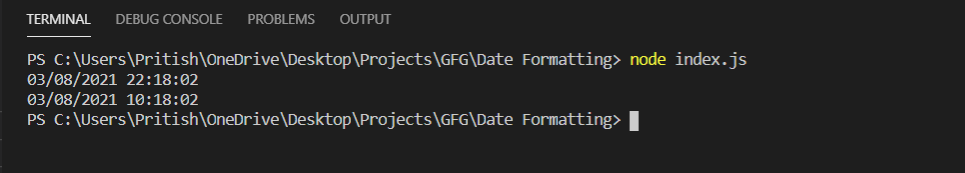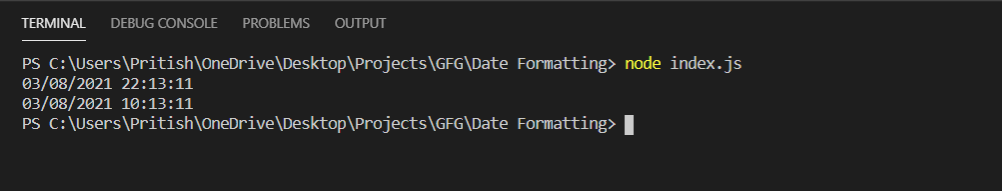Open In App

# How to format the current date in MM/DD/YYYY HH:MM:SS format using Node.js?

The current date can be formatted by using the Nodejs modules like Date Object or libraries like moment.js, dayjs

Method 1: Using Node.js Date Object

The JavaScript Date Object can be used in the program by using the following command.

`const date = new Date();`

Now on this variable date, we can apply methods to obtain various results according to the requirement. Some methods are:

• getDate(): The method returns the current date.
• getMonth(): The method returns current month.
• getFullYear(): The method returns the current year.
• getHour(): The method returns the current hour in 24-hour format.
• getMinutes(): The method returns minutes of the current hour.
• getSeconds(): The method returns seconds of the current minute.

Below is the implementation of the program:

• In the object format dd is current day, mm is month, yyyy is year, HH is hour in 24-hour format, hh is hour in 12-hour format, MM is minutes, SS is seconds.
• The function formatData() takes an input and checks if it is greater than 9 or not. If it is greater than 10 it will return it without any change but if it is less than 10 then it will append a 0 in front of the input.
• The function formatHour() takes hours as input and converts it according to the 12-hour clock.
• The functions format24Hour() and format12Hour() prints the date in format MM/DD/YYYY HH:MM:SS in 24-hour and 12-hour format respectively.

## index.js

 `const date = ``new` `Date();`` ` `// Function to convert``// single digit input``// to two digits``const formatData = (input) => {``  ``if` `(input > 9) {``    ``return` `input;``  ``} ``else` `return` ``0\${input}`;``};`` ` `// Function to convert``// 24 Hour to 12 Hour clock``const formatHour = (input) => {``  ``if` `(input > 12) {``    ``return` `input - 12;``  ``}``  ``return` `input;``};`` ` `// Data about date``const format = {``  ``dd: formatData(date.getDate()),``  ``mm: formatData(date.getMonth() + 1),``  ``yyyy: date.getFullYear(),``  ``HH: formatData(date.getHours()),``  ``hh: formatData(formatHour(date.getHours())),``  ``MM: formatData(date.getMinutes()),``  ``SS: formatData(date.getSeconds()),``};``const format24Hour = ({ dd, mm, yyyy, HH, MM, SS }) => {``  ``console.log(`\${mm}/\${dd}/\${yyyy} \${HH}:\${MM}:\${SS}`);``};``const format12Hour = ({ dd, mm, yyyy, hh, MM, SS }) => {``  ``console.log(`\${mm}/\${dd}/\${yyyy} \${hh}:\${MM}:\${SS}`);``};`` ` `// Time in 24 Hour format``format24Hour(format);``// Time in 12 Hour format``format12Hour(format);`

Run index.js file using below command:

`node index.js`

Output:Method 2: Using Moment.js Library.

## index.js

 `const moment = require(``"moment"``);`` ` `// 24 Hour format``console.log(moment().format(``"MM/DD/YYYY HH:mm:ss"``));``// 12 Hour format``console.log(moment().format(``"MM/DD/YYYY hh:mm:ss"``));`

Run index.js file using below command:

`node index.js`

Output: